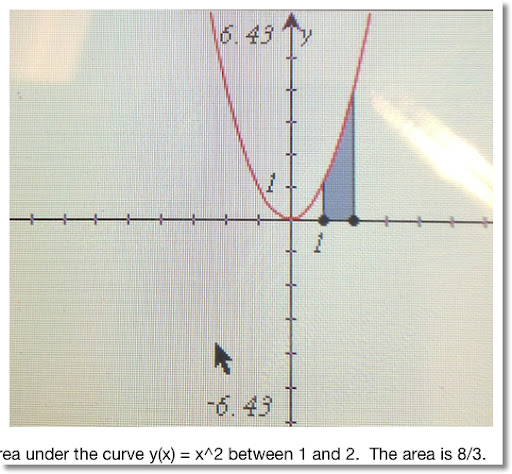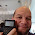## Monday, April 16, 2012

### Calculus Revisited #10: Integration Basics

Welcome to Part 10 of our 21 Calculus Revisited series. Today, we enter the world of Integration.

A function f(x) has an antiderivative F(x) if the following is true:

∫ f(x) dx = F(x) and d/dx F(x) = f(x)

Indefinite Integral: Finding a symbolic integral of f(x) to describe all the antiderivatives of f(x).

In a calculus course, if F(x) is the antiderivative of f(x), then

∫ f(x) dx = F(x) + C

where C is a numerical constant. Calculators with CAS (computer algebraic system) capabilities usually do not include the C when figuring out indefinite integrals. It is not technically wrong, the CAS system assumes that C = 0, most of the time.

Definite Integral: An integral that has a limit from a
to b (usually a < b, but not always) which produces a numerical answer. The most famous example of a definite integral is the area under the curve f(x) from a to b.

By the Fundamental Theorem of Calculus,

b
∫ f(x) dx = F(b) - F(a)
aTable of Integrals

Addition Rule:
∫ f(x) + g(x) dx = ∫ f(x) dx + ∫ g(x) dx

∫ a dx = a * x (a is a constant)
∫ x^n dx = (x^(n+1)) / (n+1)
∫ 1/x dx = ln x
∫ e^x dx = e^x
∫ sin x dx = -cos x
∫ cos x dx = sin x
∫ tan x dx = -ln |cos x|
∫ asin x dx = x asin x + √(1 - x^2)
∫ acos x dx = x acos x - √(1 - x^2)
∫ atan x dx = x atan x - ln(x^2 + 1)/2
∫ 1/√(1 - x^2) dx = asin x
∫ 1/(1 + x^2) dx = atan x
∫ 1/√(x^2 - 1) dx = ln(√(x^2 - 1) + x)
∫ a^x dx = a^x/(ln a)

Problems
Indefinite Integrals

1. ∫ 2x^2 + x - 1 dx

∫ 2x^2 + x - 1 dx
= ∫ 2x^2 dx + ∫ x dx - ∫ 1 dx
= 2/3 * x^3 + C + x^2/2 + C - x + C
C just symbolizes a general constant, do not worry if it is 3C or just C in this case
= 2/3 * x^3 + x^2/2 - x + C

In the future, for indefinite integral problems, we will just add the C at the end.

2. ∫ 1/x^2 - 1/x dx
= ∫ x^(-2) dx - ∫ 1/x dx
= -1/x - ln x + C

3. ∫ 2 * 3^x dx

Note: ∫ a * f(x) dx = a * ∫ f(x) dx. Know this - it will come in handy a lot.

∫ 2 * 3^x dx
= 2 * ∫ 3^x dx
= 2 * (3^x)/ln 3 + C

Definite Integrals

Hint: When using a calculator to calculate definite integrals - as a general rule, always have your calculator in Radians mode.

4.

1
∫ 1/(√(1 - x^2) dx
0

Use the Fundamental Theorem of Calculus to assist us with these type of problems.

f(x) = 1/(√(1 - x^2)
F(x) = asin x

1
∫ 1/(√(1 - x^2) dx
0

= asin 1 - asin 0
= π/2 - 0
= π/2

Hint: Do not worry about the "C" with definite integrals, they cancel out!

b
∫ f(x) dx
a

= F(b) + C - (F(a) + C)
= F(b) + C - F(a) - C
= F(b) - F(a)

5.

2
∫ 2 * e^x dx
0

2
= ∫ e^x dx
0

= 2 (e^2 - e^0)
= 2 (e^2 - 1) ≈ 12.77811

( ∫ e^x dx = e^x )

Limits do not have to be constants. Check out the next problem.

6.

t
∫ cos x dx
0

= sin(t) - sin 0
= sin(t)

( ∫ cos x dx = sin x)

Initial Value Problems - Basically, figure out what C is.

7. Find the antiderivative of f(x) = 2x + 5 if the antiderivative passes the point (0, 1).

We are faced with two tasks:
1. Find F(x) in general
2. Find the specific F(x) knowing F(0) = 1. This is where we solve for C.

∫ f(x) dx = F(x) + C

∫ 2x + 5 dx
= x^2 + 5x + C

With F(0) = 1 (going through point (0,1)): (x = 0, y = 1)

1 = (0)^2 + 5 * (0) + C
1 = 0 + C
1 = C

The final answer is F(x) = x^2 + 5x + 1

8. The slope of the function y(x) is described by the function sin x. It is known that y(x) passes through the point (1/2, 1/2). Find y(x).

The slope of the function y(x) is the derivative of y(x), or y'(x).

Then ∫ y'(x) dx = y(x)

And
∫ sin x dx = - cos x + C
Almost there, we have to find what C is. Use the point (1/2, 1/2) to help.
1/2 = - cos(1/2) + C
1/2 + cos(1/2) = C
We can conclude that
y(x) = cos x + (1/2 + cos(1/2)) ≈ cos x + 1.37758

We have just started with integrals. The next four parts will deal with techniques of integration.

See you soon, Eddie

This blog is property of Edward Shore. © 2012

#### 3 comments:

1.I really needed these formulas of integration.These formulas very helpful in solving the calculus problems of high school's mathematics.
Integration by Substitution

2.Wilson, Thank you, I am glad this helped.

3.### Update: HP Prime Connectivity Kit and Emulator

Update: HP Prime Connectivity Kit and Emulator The HP Prime Connectivity Kit and Emulator have been updated.  The version number is 14592 ...